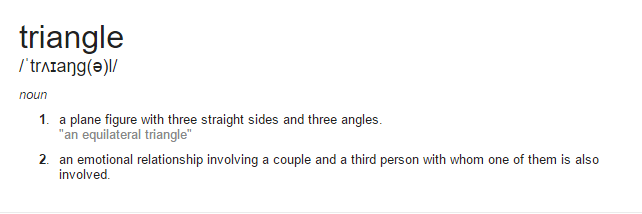# Java Program To Calculate Area Of Triangle – 5 Ways

Java program to calculate the area of a triangle when three sides are given or normal method. Calculating the area of a triangle is quite simple if you know the basics of java programming. If you were at newbie level to learn the Java programming then check out the following tutorial so that you will get an idea.

The following program has been written in three different ways using the static method, basic formula when three sides are given, user-defined method, using a constructor with sample outputs.

What is a triangle?

Triangle, is plane-sided or which looks like a pyramid, have three sides and three angles in whatever length or angle it may be, but sum-it-up to 180 degrees.What is the formula to find the calculate the area of a triangle?

The formula is varied for different types of triangle, but the most common formula that was used as

(Height X Base /2 )

Consider the following program as a sample method – 1, there. There were more than 2 methods here listed below check it out. Moreover, if you have any doubts related to this section, then do comment at the end of the post we are glad to help you out.

## 5 Different Ways To Find Area Of Triangle

Sample method -1 # To find or calculate the area of a triangle #

Output :

Online Execution & Compiler Tool To Calculate Area

If you were new to java programming, then check out the below explanation about how the above java code works. If you knew the basic level of programming, then skip it and move on to second sample method with online execution and compiler too.

1)

– Type which passes the primitive types and strings.

2)

– In the real world, you’ll often find many individual objects all of the same kind. There may be thousands of other bicycles in existence, all of the same make and model.

Each bicycle was built from the same set of blueprints and therefore contains the same components. In object-oriented terms, we say that your bicycle is an instance of the class of objects known as bicycles.

A class is a blueprint from which individual objects are created.

3)

– the Main function, the main program will start reading the values from here. Consider it has a starting function of the main block.

4)

– Scanner read the values from the main java.util.Scanner; or consider it has read the inputs values, whereas system.in is to read the values from your system or device.

5)

– Displays whatever you were written.

6)

-is a datatype.

7)

– The formula to find the area of a triangle.

8)

– The output will be displayed here.

### 2. Java Program Calculate Area Of Triangle Using Constructor

There you go another method, using the constructor with sample output example.

output:

3. Using User-Defined Method

Another method, using user-defined method or function.

output:

#### 4.Using when three sides are given

Basically, In order to calculate the area, you need to find out the Height of the triangle. If you don’t know the height or you may have no idea how to find out the height of the triangle, then you can use the below program to calculate the area of a triangle.

When three sides are given we have used the following formula: only when three sides are given.

s(s-a)(s-b)(s-c)

OutPut :

Online Execution Tool for the above java code:

Above one is another simple method to find the area of the triangle here the formula is :

s(s-a)(s-b)(s-c)

Where the value of S is {( a+b+c)/2} and the loop method as ” if((a+b)>c && (a+c)>b && (b+c)>a) ”

The above example, we have used the datatype “Int”. It’s all up to you, you can even use either float or double.

Why ” Double ” but not ” Int”?

Every type of data types has significant memory storage capacity, for instance in, the Int can store the memory up to 4 bytes, whereas ” Double ” Can store the memory values up to 8-bytes.